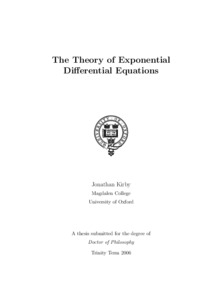# The Theory of Exponential Differential Equations

Kirby, J (2006) The Theory of Exponential Differential Equations. Doctoral thesis, Magdalen College, University of Oxford.Preview PDF Download (31kB) | PreviewPreview PDF Download (673kB) | Preview

## Abstract

This thesis is a model-theoretic study of exponential differential equations in the context of differential algebra. I define the theory of a set of differential equations and give an axiomatization for the theory of the exponential differential equations of split semiabelian varieties. In particular, this includes the theory of the equations satisfied by the usual complex exponential function and the Weierstrass p-functions. The theory consists of a description of the algebraic structure on the solution sets together with necessary and sufficient conditions for a system of equations to have solutions. These conditions are stated in terms of a dimension theory; their necessity generalizes Ax’s differential field version of Schanuel’s conjecture and their sufficiency generalizes recent work of Crampin. They are shown to apply to the solving of systems of equations in holomorphic functions away from singularities, as well as in the abstract setting. The theory can also be obtained by means of a Hrushovski-style amalgamation construction, and I give a category-theoretic account of the method. Restricting to the usual exponential differential equation, I show that a “blurring” of Zilber’s pseudo-exponentiation satisfies the same theory. I conjecture that this theory also holds for a suitable blurring of the complex exponential maps and partially resolve the question, proving the necessity but not the sufficiency of the aforementioned conditions. As an algebraic application, I prove a weak form of Zilber’s conjecture on intersections with subgroups (known as CIT) for semiabelian varieties. This in turn is used to show that the necessary and sufficient conditions are expressible in the appropriate first order language.

Item Type: Thesis (Doctoral) Faculty of Science > School of Mathematics Jonathan Kirby 18 Mar 2011 14:41 20 Oct 2011 10:19 https://ueaeprints.uea.ac.uk/id/eprint/20122View Item